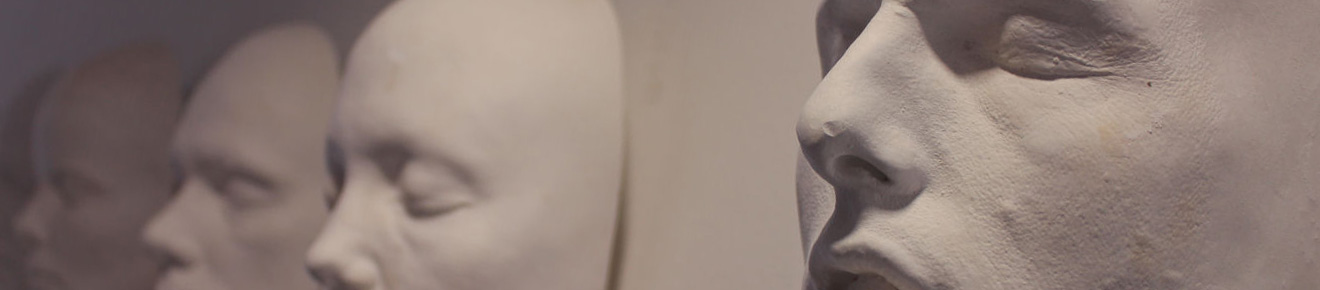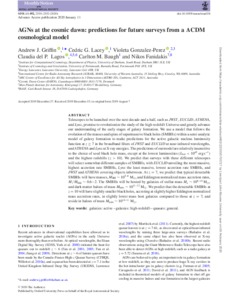# AGNs at the cosmic dawn: predictions for future surveys from a $Λ$CDM cosmological model

Griffin, AJ, Lacey, CG, Gonzalez-Perez, V, Lagos, CDP, Baugh, CM and Fanidakis, N (2020) AGNs at the cosmic dawn: predictions for future surveys from a $Λ$CDM cosmological model. Monthly Notices of the Royal Astronomical Society, 492 (2). pp. 2535-2552. ISSN 0035-8711Preview
Text
AGNs at the cosmic dawn predictions for future surveys from a $Λ$CDM cosmological model.pdf - Published Version

Telescopes to be launched over the next decade-and-a-half, such as JWST, EUCLID, ATHENA and Lynx, promise to revolutionise the study of the high redshift Universe and greatly advance our understanding of the early stages of galaxy formation. We use a model that follows the evolution of the masses and spins of supermassive black holes (SMBHs) within a semi-analytic model of galaxy formation to make predictions for the Active Galactic Nucleus (AGN) luminosity function at $z\geq7$ in the broadband filters of JWST and EUCLID at near-infrared wavelengths, and ATHENA and Lynx at X-ray energies. The predictions of our model are relatively insensitive to the choice of seed black hole mass, except at the lowest luminosities ($L_{\mathrm{bol}}<10^{43}\mathrm{ergs^{-1}}$) and the highest redshifts ($z>10$). We predict that surveys with these different telescopes will select somewhat different samples of SMBHs, with EUCLID unveiling the most massive, highest accretion rate SMBHs, Lynx the least massive, lowest accretion rate SMBHs, and JWST and ATHENA covering objects inbetween. At $z=7$, we predict that typical detectable SMBHs will have masses, $M_{\mathrm{BH}}\sim10^{5-8}M_{\odot}$, and Eddington normalised mass accretion rates, $\dot{M}/\dot{M}_{\mathrm{Edd}}\sim0.6-2$. The SMBHs will be hosted by galaxies of stellar mass $M_{\star}\sim10^{8-10}M_{\odot}$, and dark matter haloes of mass $M_{\mathrm{halo}}\sim10^{11-12}M_{\odot}$. We predict that the detectable SMBHs at $z=10$ will have slightly smaller black holes, accreting at slightly higher Eddington normalised mass accretion rates, in slightly lower mass host galaxies compared to those at $z=7$, and reside in haloes of mass $M_{\mathrm{halo}}\sim10^{10-11}M_{\odot}$.View Item